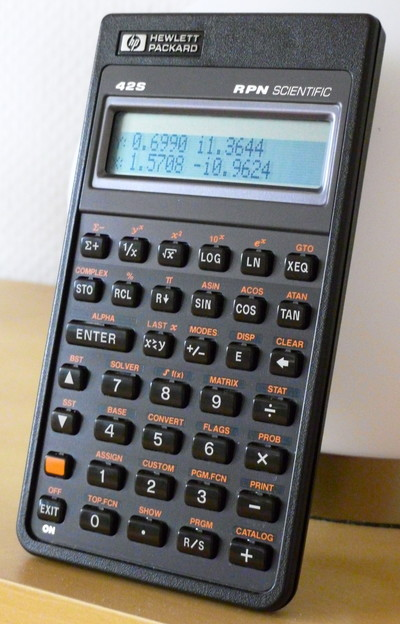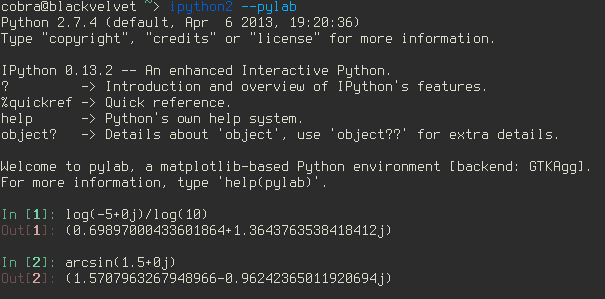RPN

I bought my first electronic calculator when I enrolled in physics at the university (they were usually not permitted at school at that time). In the late 70s and early 80s, there were only two serious contenders in the calculator market, Hewlett Packard (HP) and Texas Instruments (TI). HP had RPN (reverse Polish notation), TI not. I found the concept fascinating, exhausted all financial resources over which I commanded, and acquired an HP-15c. Since then I'm an all-out RPN advocate. Hell, I can't even use the infix notation anymore.

The 15c served me well for many years, but got replaced by a HP-32s when I was working on my PhD. Only a year after, and on another continent, my employer presented me with a HP-42s. Since then (and that's 20 years ago), I use these two calculators day in, day out.

Here's my 42s in action. To show that it deals with complex numbers in a straightforward way, I let it calculate the logarithm of -5 and the inverse sine of 1.5:The results are correctly rounded:Every Unix system comes with an RPN calculator, and an extremely powerful one: dc (part of bc under Linux).

Here's dc in action, calculating this expression

$\frac{(1+2)(3+4)}{(5+6)(7+8)}{\small 9}-\small{ 10}$

to 20 digits. Below is the same calculation with bc. Ignoring the spaces (which are not needed on a calculator), the former requires 19 keystrokes, the latter 30.On a computer, I use the number block on the right of the keyboard to perform such small calculatons. Depending on the distribution, the ',' key may return a comma for a German keyboard layout, but I need a decimal point there as neither bc nor dc recognize numbers such as 15,99.

The command

xmodmap -e "keycode 91 = period period"

changes the assignment for this particular key. For a permanent change, issue afterwards

xmodmap -pke | grep 91 > ~/.Xmodmap